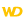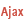• Use conditions
• Multi-selection Table control
• Horizontal Table control
• Handling errors
WINDEVWEBDEVWINDEV MobileOthersMoves a row or swaps two rows in a Table control. To move the columns in a Table control, use <Table>.MoveColumn.
Example
// Swap the current row and row 5
TABLE_Table1.MoveLine(SelectedLine, 5, amSwap)
// Move the current row to row 3
TABLE_Table1.MoveLine(SelectedLine, 3, amMove)
// Swap the current row with the next row
TABLE_Table1.MoveLine(SelectedLine, 5, amNext, amSwap)
Syntax

Moving to a specific position

<Result> = <Table control>.MoveLine(<Index of row to move> , <Destination row index> [, <Operation to perform>])
<Result>: Integer
New index of the row that was moved.
<Table control>: Control name
Name of the Table control to be used.
<Index of row to move>: Integer
Position of the row to move. This position must be between 1 and the number of rows in the Table control (returned by the <Table>.Count function or the Count property, for example).
To move the row that is currently selected, use the rowSelected constant.
<Destination row index>: Integer
New row position. This position must be between 1 and the number of rows in the Table control (returned by the <Table>.Count function or the Count property, for example). A fatal error occurs if this parameter is not valid.
To handle the row that is currently selected, use the rowSelected constant.
<Operation to perform>: Optional Integer constant (or combination of constants)
Change row position:
 amDisplayDestination The moved (or swapped) row is displayed in the Table control. If necessary, the Table control is automatically scrolled to show the row at its new position. The selected row (selection bar) does not change.This constant can be combined with the amMove and amSwap constants.Table in Ajax mode: The moved row will always be displayed at the top of the Table control. amMove(default value) Moves the row to the index of the destination row. amSwap Swaps the position of the source and destination row.Moving relatively to the current position

<Result> = <Table control>.MoveLine(<Index of row to move> , <Relative move> [, <Operation to perform>])
<Result>: Integer
New index of the row that was moved.
<Table control>: Control name
Name of the Table control to be used.
<Index of row to move>: Integer
Position of the row to move. This position must be between 1 and the number of rows in the Table control (returned by the <Table>.Count function or the Count property, for example).
To handle the row that is currently selected, use the rowSelected constant.
<Relative move>: Integer constant
Moves or swaps a row to a position relative to the index of that row.
 amFirst Moves the row to the first position. amLast Moves the row to the last position. amNext Moves the row one position downwards. amNextPage Moves the row one page to the bottom (a page corresponds to the number of rows that can be simultaneously displayed in the Table control). amPrevious Moves the row one position upwards. amPreviousPage Moves the row one page to the top (a page corresponds to the number of rows that can be simultaneously displayed in the Table control).

If this parameter is invalid (e.g., if the amPrevious constant is used on the first row of the Table control), the row is moved "to the nearest" position.
<Operation to perform>: Optional Integer constant (or combination of constants)
Change row position:
 amDisplayDestination The modified row is displayed in the Table control. If necessary, the Table control is automatically scrolled to show the row at its new position. The selected row (selection bar) does not change.This constant can be combined with the amMove and amSwap constants.Table in Ajax mode: The modified row will always be displayed at the top of Table control. amMove(default value) Moves the row to the index of the destination row. amSwap Swaps the position of the source and destination row.
Remarks

Use conditions

<Table>.MoveLine can be used on:
• a Table control populated programmatically.
• a Table control with in-memory data source.
•a horizontal Table control.
•a Table control in "Server" or "Server + AJAX" mode.
•a Table control in "Browser" mode.
This function cannot be used on TreeView Table controls and on Table controls based on a data file (direct access).
Remarks: Moving a row according to the type of control:

Multi-selection Table control

If <Table>.MoveLine is used on a multi-selection Table control:
• when moving a row (amMove constant): only the first selected row will be moved.
• when swapping rows (amSwap constant): only the first row will be swapped.
• the rowSelected constant cannot be used. Otherwise, a fatal error occurs and no row is moved.Horizontal Table control

If <Table>.MoveLine is called on a horizontal Table control, columns will be moved instead of rows.
To move the rows in a horizontal Table control, use <Table>.MoveColumn.

Handling errors

Caution: <Table>.MoveLine returns no error code. To determine if this function has generated an error, use ErrorInfo with the errMessage constant.ErrorInfo is not available.
Component: wd280obj.dll
Minimum version required
• Version 23